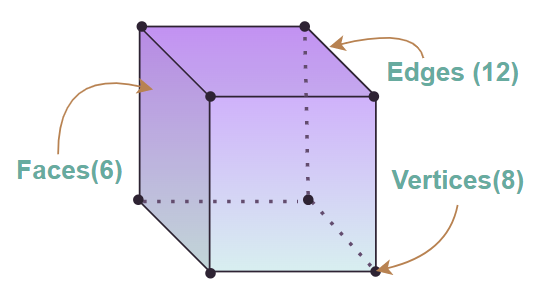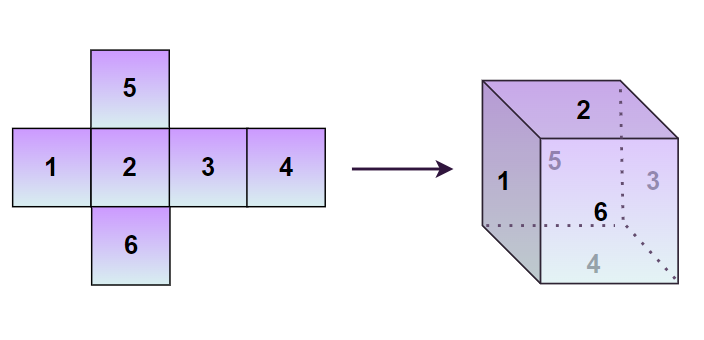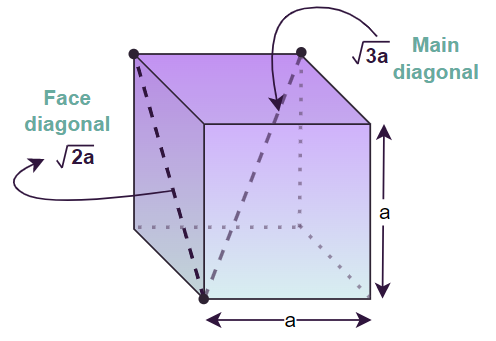# Cube – Definition, Shape & Formula

Cube is a solid or hollow three-dimensional form of a square that has six square faces, eight vertices, and twelve edges. Some examples of a cube that we see regularly are sugar cubes, ice cubes, Rubik’s cubes, etc. The length, breadth, and height of a cube are the same as each face of a cube is a square.

A cube is also known as an equilateral cuboid, a square parallelepiped, or a right rhombic hexahedron and is one of the five platonic solids.

In this article, we will discuss what is a cube, cube shape, formulas related to cubes, properties, and examples.

## What is a Cube?

Cube is a 3D solid shape with six square faces with all 12 sides of equal length. A cube can be considered a special cuboid where all the length, breadth, and height are equal. A cube is a special case of a square prism having six square faces, eight vertices, and twelve edges.

We see various shapes in our daily lives that resemble the cube. Such as a 3×3 Rubik’s Cube a puzzle famous among many, is an example of a cube. The image added below shows a cube along with its faces, edges, and vertices.

### Cube Definition

A cube is a three-dimensional geometric shape characterized by six equal square faces, twelve equal edges, and eight vertices (corners). Each of its six faces is a square, and all the angles between the faces are right angles (90 degrees)Studying the above figure, we conclude that the two faces of the cube have a common boundary called the edge of the cube and there are twelve (12) edges of the cube, similarly on observing the figure closely we conclude that a cube has six (6) faces and eight (8) vertices.

## Cube Shape

Cube shape is one of the fundamental shapes of mathematics and is observed very often in our daily lives. We can assume the cube is a polyhedron with equal length, breadth, and height. It can be easily stacked on one over another without leaving any spaces. We say about a cube that,

• It has Twelve (12) Edges
• It has Six (6) Faces
• It has Eight (8) Vertices

We see various types of figures in our daily life that are shaped like cubes that include, boxes, ice cubes, sugar cubes, etc.

## Properties of Cube

A cube is a 3D figure with equal dimensions having various properties. Some of the properties of the cube are,

• All the faces of a cube are square-shaped. Hence the length, breadth, and height of a cube are equal.
• The angle between any two faces of a cube is a right angle, i.e., 90°.
• Each face of a cube meets the other four faces.
• Three edges and three faces of a cube meet at a vertex.
• The opposite edges of a cube are parallel to each other.
• The faces or planes of a cube opposite to each other are parallel.

## Net of Cube

A cube is a 3-D figure and a figure in 2-D that can be folded easily to form the cube is called the net of a cube. Thus, we can say that the two-dimensional form of a cube that can be folded to form a three-dimensional form is called a net of a cube. There are various ways to unfold a cube, i.e. a cube can have various nets one of the nets of the cube is discussed in the image below,## Cube Formula

There are various formulas that are helpful to find various dimensions of the cube, that include the length of its diagonal, its surface area, its volume, etc. various cube formulas discussed in the article are,

• Diagonal of Cube
• Surface Area of Cube
• Volume of Cube

Now let’s learn about these formulas in detail.

## Diagonal of Cube

The diagonal of a cube is the line segment that joins the opposite vertices of the cube. A cube has two types of diagonals, i.e., a face diagonal and a main diagonal. A face diagonal is a line that joins the opposite vertices of the face of a cube and is equal to the square root of two times the length of the side of a cube. As the cube has six faces, it has a total of 12 face diagonals. The formula to calculate the face diagonal of the cube is,

Length of Face Diagonal of Cube = √2a units

where a is the length of the side of a cube

While the main diagonal is the line segment that joins the opposite vertices, passing through the center of the cube, and is equal to the square root of three times the length of the side of a cube. A cube has a total of four main diagonals.

Length of Main Diagonal of Cube = √3a units

where a is the length of the side of a cube

The below image represents the main diagonal and the face diagonal of the cube.## Surface Area of Cube

The area of any object is the space occupied by all the surfaces of that object. It can be defined as the total surface available for the painting. A cube has six faces and so its surface area is calculated by finding the area of the individual face and finding its sum.

There are two types of surface area associated with a cube that are mentioned below,

• Lateral Surface Area of the Cube, also called LSA of the Cube.
• Total Surface Area of Cube, also called TSA of Cube.

### Lateral Surface Area of Cube

The Lateral Surface Area of a cube is the sum of the areas of all the faces of a cube, excluding its top and bottom. In simple words, the sum of all four side faces of a cube is the lateral surface area of a cube. It is measured in square units such as (units)2, m2, cm2, etc.

The formula for the lateral surface of a cube is

Lateral Surface Area of Cube = 4a2

where
a is length of side of a cube

### Total Surface Area of Cube

The Total Surface Area of a cube is the space occupied by it in three-dimensional space and is equal to the sum of the areas of all its sides. It is measured in square units such as (units)2, m2, cm2, etc.

The formula for the total surface of a cube is

Total Surface Area of Cube = 6a2

where
a is the length of the side of a cube.

## Volume of Cube

The volume of a cube is the amount of space enclosed by the cube. It is usually measured in terms of cubic units. It is measured in cube units such as (units)3, m3, cm3, etc.

The formula for the volume of a cube is

Volume of a Cube = a3

where
a is the length of the side of a cube

We can also calculate the volume of the cube if its diagonal is given, by using the formula,

Volume of Cube = (√3d3)/9

where
d is the length of the main diagonal of the cube.

## Solved Example on Cube Formula

Example 1: Find the total surface area of a cube if the length of its side is 8 units.

Solution:

Given,

• Length of side of Cube (a) = 8 units

We know that,

Total Surface Area of Cube (TSA) = 6a2

TSA = 6 × (8)2

= 6 × 64

= 384 square units.

Hence, the surface area of the cube = 384 square units.

Example 2: Find the volume of a cube if the length of its side is 5.5 inches.

Solution:

Given,

• Length of side of Cube (a) = 5.5 inches.

We  know that,

Volume of Cube (V) = a3

V = (5.5)3

= 166.375‬ cubic inches

Hence, the volume of the cube is 166.375‬ cubic inches.

Example 3: Find the length of the diagonal of a cube and its lateral surface area if the length of its side is 6 m.

Solution:

Given,

• Length of side of Cube (a) = 6 m

We know that,

Length of Diagonal of Cube(l) = √3 a

l = √3 × 6

= 6√3 m

Lateral Surface Area of Cube (LSA) = 4a2

LSA = 4 × (6)2

= 4 × 36

= 144 m2

Hence, the length of the diagonal is 6√3 m, and its lateral surface area is 144 square meters.

Example 4: Determine the length of the diagonal of the cube if the volume of the cube is 91.125 cm3.

Solution:

Given,

Volume of the cube (V) = 91.125 cm3

Let length of side of a cube be “s”

We have,

Volume of Cube = s3

s3 = 91.125

s =  ∛(91.125)

= 4.5 cm

Length of diagonal of a Cube(l) = √3 s

l = √3 × 4.5 = 4.5√3 cm.

Hence, the length of the diagonal is 4.5√3 cm

Example 5: Determine the volume of the cube if its total surface area is 54 square units.

Solution:

Given,

Total Surface Area of Cube = 54 square units.

Let length of side of a cube be “a”

We have,

Surface Area of Cube (A) = 6a2

6a2 = 54

a2 = 54/6 = 9

a = √9 = 3 units

We know that,

Volume of Cube (V) = a3

V = (3)3

= 27 cubic units

Hence, the volume of the cube = 27 cubic units

Example 6: Find the lateral surface area and the total surface area of a cube if the length of its diagonal is 5√3 units.

Solution:

Given,

Length of Diagonal of Cube = 5√3 units

Let length of side of a cube be “a”

We have,

Length of Diagonal of Cube = √3 a

5√3 = √3 a

a = 5√3/√3 = 5 units

Lateral Surface Area of Cube (LSA) = 4a2

LSA = 4 × (5)2

= 4 × 25 = 100 square units.

Total Surface Area of Cube (TSA) = 6a2

TSA = 6 × (5)2

= 6 × 25

= 150 square units.

Hence, the lateral surface area of a cube is100 square units, and the total surface area is 150 square units.

Example 7: Find the length of the side of a cube if its lateral surface area is 196 square inches.

Solution:

Given,

Lateral Surface Area of Cube = 196 square inches

Let length of side of Cube be “a”

We have,

Lateral Surface Area of Cube (LSA) = 4a2

196 = 4a2

a2 = 196/4 = 49

a = √49 = 7 inches

Hence, length of side of cube is 7 inches

## FAQs on Cube

### Q1: What is a Cube?

A cube is a three-dimensional figure with equal length, breadth and height. It is formed by taking six square faces of equal sides together.

### Q2: What is the difference between a Cube and a Cuboid?

Cube and Cuboid both are three-dimensional figures and the basic difference between them is, that in a cube all the length, breadth and height are equal, whereas in cuboid the length breadth and height of the figure are different.

### Q3: What is the formula to calculate the Surface Area of a Cube?

The Surface Area of a Cube is calculated using the formula,

• Lateral Surface Area of Cube (LSA) = 4a2
• Total Surface Area of Cube (TSA) = 6a2

where a is the side length of the cube.